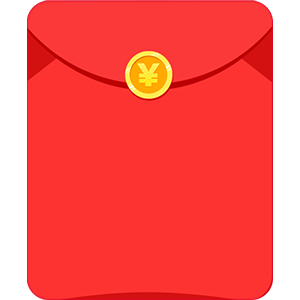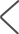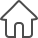⒉点A为直线y=2x+2上的一点，点A到两坐标轴的距离相等，那A点坐标为────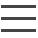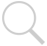×

9-99元的随机现金红包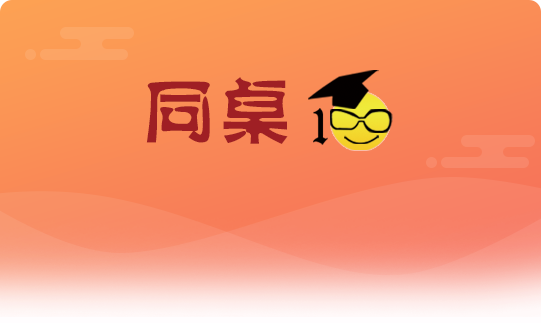```⒈一次函数Y1=K1x-4与正比例函数Y2=K2x的图像都过点（2,1）
①分别求出这两个函数的解析式
②求这两个函数图象与X轴围成的三角型面积 （画图)

⒉点A为直线y=2x+2上的一点，点A到两坐标轴的距离相等，那A点坐标为────

3.已知一次函数y=2/3x+m与y=-1/2x+n的图像交于点A（-2.0）且与Y轴的焦点分别是B,C求三角形ABC的面积```

```解:

Y=2.5X-4
Y=0.5X

A(8/5,0)
O(0,0)

= 0.5×BC×OC-0.5×AC×BC
= 0.5×1×2-0.5×0.4×1
= 1-0.02=0.98
```
```两种情况
1.   x=y
x=-2x+2
x=2/3,y=2/3
2.   x=-y
x=2,y=-2

```
```一次函数y=2/3x+m和y=-1/2x+n的图像都经过点A(-2,0)，

y=2/3x+4/3 令x=0得B（0,4/3)
y=-1/2x-1  令x=0得C（0,-2）

S=0.5*|BC|*2=10/3
```